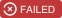Latest 1.5.0 https://github.com/TakeScoop/SwiftyRSA MIT ios 8.3, tvos 9.2, watchos 2.2, requires ARC Security Scoop

Maintainer(s): @ldiqualPublic key RSA encryption in Swift.

SwiftyRSA is used in the Scoop iOS app to encrypt driver license numbers before submitting them to Checkr through our API.

## Installation

### Swift 3.2 / 4.0+

With Cocoapods:

``pod 'SwiftyRSA'``

With Carthage:

``github "TakeScoop/SwiftyRSA"``

### Swift 2.3

The `swift-2.3` branch is built out of SwiftRSA 0.4.0 and is not actively maintained. If you’d like to use the latest features of SwiftyRSA, please use swift 3.0.

``pod 'SwiftyRSA', :git => '[email protected]:TakeScoop/SwiftyRSA.git', :branch => 'swift-2.3'``

### Objective-C

``pod 'SwiftyRSA/ObjC'``

## Quick Start

### Encrypt with a public key

``````let publicKey = try PublicKey(pemNamed: "public")
let clear = try ClearMessage(string: "Clear Text", using: .utf8)
let encrypted = try clear.encrypted(with: publicKey, padding: .PKCS1)

// Then you can use:
let data = encrypted.data
let base64String = encrypted.base64String``````

### Decrypt with a private key

``````let privateKey = try PrivateKey(pemNamed: "private")
let encrypted = try EncryptedMessage(base64Encoded: "AAA===")
let clear = try encrypted.decrypted(with: privateKey, padding: .PKCS1)

// Then you can use:
let data = clear.data
let base64String = clear.base64String
let string = clear.string(using: .utf8)``````

### Create a public/private key representation

#### With a DER file

``````let publicKey = try PublicKey(derNamed: "public")
let privateKey = try PrivateKey(derNamed: "private")``````

#### With a PEM file

``````let publicKey = try PublicKey(pemNamed: "public")
let privateKey = try PrivateKey(pemNamed: "private")``````

#### With a PEM string

``````let publicKey = try PublicKey(pemEncoded: str)
let privateKey = try PrivateKey(pemEncoded: str)``````

#### With a Base64 string

``````let publicKey = try PublicKey(base64Encoded: base64String)
let privateKey = try PrivateKey(base64Encoded: base64String)``````

#### With data

``````let publicKey = try PublicKey(data: data)
let privateKey = try PrivateKey(data: data)``````

#### With a SecKey

``````let publicKey = try PublicKey(reference: secKey)
let privateKey = try PrivateKey(reference: secKey)``````

### Encrypt with a public key

``````let str = "Clear Text"
let clear = try ClearMessage(string: str, using: .utf8)
let encrypted = try clear.encrypted(with: publicKey, padding: .PKCS1)

let data = encrypted.data
let base64String = encrypted.base64Encoded``````

### Decrypt with a private key

``````let encrypted = try EncryptedMessage(base64Encoded: base64String)
let clear = try encrypted.decrypted(with: privateKey, padding: .PKCS1)

let data = clear.data
let base64String = clear.base64Encoded
let string = try clear.string(using: .utf8)``````

### Sign with a private key

SwiftyRSA can sign data with a private key. SwiftyRSA will calculate a SHA digest of the supplied `String`/`Data` and use this to generate the digital signature.

``````let clear = try ClearMessage(string: "Clear Text", using: .utf8)
let signature = clear.signed(with: privateKey, digestType: .sha1)

let data = signature.data
let base64String = signature.base64String``````

### Verify with a public key

SwiftyRSA can verify digital signatures with a public key. SwiftyRSA will calculate a digest of the supplied `String`/`Data` and use this to verify the digital signature.

``````let signature = try Signature(base64Encoded: "AAA===")
let isSuccessful = try clear.verify(with: publicKey, signature: signature, digestType: .sha1)``````

### Create a public/private RSA key pair

``````let keyPair = SwiftyRSA.generateRSAKeyPair(sizeInBits: 2048)
let privateKey = keyPair.privateKey
let publicKey = keyPair.publicKey``````

### Export a key or access its content

``````let pem = try key.pemString()
let base64 = try key.base64String()
let data = try key.data()
let reference = key.reference
let originalData = key.originalData``````

## Create public and private RSA keys

Use `ssh-keygen` to generate a PEM public key and a PEM private key. SwiftyRSA also supports DER public keys.

``````\$ ssh-keygen -t rsa -f ~/mykey -N ''
\$ cat ~/mykey > ~/private.pem
\$ ssh-keygen -f ~/mykey.pub -e -m pem > ~/public.pem``````

Your keys are now in `~/public.pem` and `~/private.pem`. Don’t forget to move `~/mykey` and `~/mykey.pub` to a secure place.

## Under the hood

To enable using public/private RSA keys on iOS, SwiftyRSA uses a couple techniques like X.509 header stripping so that the keychain accepts them.

When encrypting using a public key:

– If the key is in PEM format, get rid of its meta data and convert it to Data
– Strip the public key X.509 header, otherwise the keychain won’t accept it
– Add the public key to the keychain, with a random tag
– Get a reference on the key using the key tag
– Use `SecKeyEncrypt` to encrypt a `ClearMessage` using the key reference and the message data.
– Store the resulting encrypted data to an `EncryptedMessage`
– When the key gets deallocated, delete the public key from the keychain using its tag

When decrypting using a private key:

– Get rid of PEM meta data and convert to Data
– Add the private key to the app keychain, with a random tag
– Get a reference on the key using the key tag
– Use `SecKeyDecrypt` to decrypt an `EncryptedMessage` using the key reference and the encrypted message data
– Store the resulting decrypted data to a `ClearMessage`
– Delete private key from keychain using tag

## Inspired from

### Latest podspec

```{
"name": "SwiftyRSA",
"version": "1.5.0",
"summary": "Public key RSA encryption in Swift.",
"description": "Encrypt with a RSA public key, decrypt with a RSA private key.",
"homepage": "https://github.com/TakeScoop/SwiftyRSA",
"authors": {
"Scoop": "[email protected]"
},
"source": {
"git": "https://github.com/TakeScoop/SwiftyRSA.git",
"tag": "1.5.0"
},
"source_files": "Source/*.{swift,m,h}",
"exclude_files": "Source/SwiftyRSA+ObjC.swift",
"frameworks": "Security",
"requires_arc": true,
"swift_version": "4.1",
"platforms": {
"ios": "8.3",
"tvos": "9.2",
"watchos": "2.2"
},
"subspecs": [
{
"name": "ObjC",
"source_files": "Source/*.{swift,m,h}"
}
]
}```